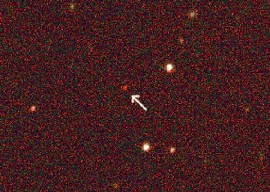Click to view animation.

The animation to the right shows schematically what the expanding universe looks like. Each galaxy, represented by a white dot, is getting farther away from every other galaxy. (You saw the same effect with dots on the balloon.)

If the universe is expanding today, as the animation shows, then at some point in the past it must have been smaller. If you go far enough back in time – if you rewind the animation far enough – then at some point the universe must have had zero size and started expanding. Astronomers call the start of expansion the “big bang.”

The “big bang” was not an explosion – it was just the time when the universe started expanding. Many modern astronomers and physicists are trying to understand what happened in the few minutes after the big bang.

## Absolute and Relative DistancesA quasar found by SDSS at redshift 5.8,

What you measured in this project was relative, not absolute, distance. Having an absolute distance means we know the value of d in light-years, meters, or some other distance.

Measuring absolute distances in astronomy is extremely difficult, but you can use the equation

`c z = H0 d`

to estimate the distance to a distant galaxy from its redshift. If you use the equation, you can go from a measurement of a galaxy’s redshift (which has no units) to its distance in light-years. But to find the distance, you need a value of the Hubble constant. If we measure c in meters per second and d in light-years, then H0 has units of meters per second per light-year.

The numerical value for the Hubble constant has been the subject of many experiments and much debate among astronomers. The currently favored value is about H0 = 0.0215 m/sec/light-year. The error associated with this number is about 10%, which reflects the uncertainty in measuring absolute distances to galaxies.

Exercise 1. If a galaxy has redshift 0.1, how far away is it in light-years? Remember that c = 3.0 x 108 m/s, and assume H0 = 0.0215 m/s/light-year.
What about a galaxy with redshift 0.2?

The Hubble constant measures the expansion rate of the universe, so the inverse of the Hubble constant (1/H0) measures the amount of time that the universe has been expanding. In other words, 1/H0, tells us the time since the Big Bang.

Exercise 2. If you assume that H0 = 0.0215 m/s/light-year, what is the age of the universe? If the age of the universe is subject to the same 10% uncertainty as the Hubble constant, what is the range of ages the universe could have?

Hints:
1 light-year = 9,460,800,000,000 kilometers
Look up the speed of light in a textbook or on the Internet

Question 5. Recent measurements have shown that the oldest stars we can see are about 12 billion years old. Are these ages consistent with the age of the universe you calculated in Exercise 2?